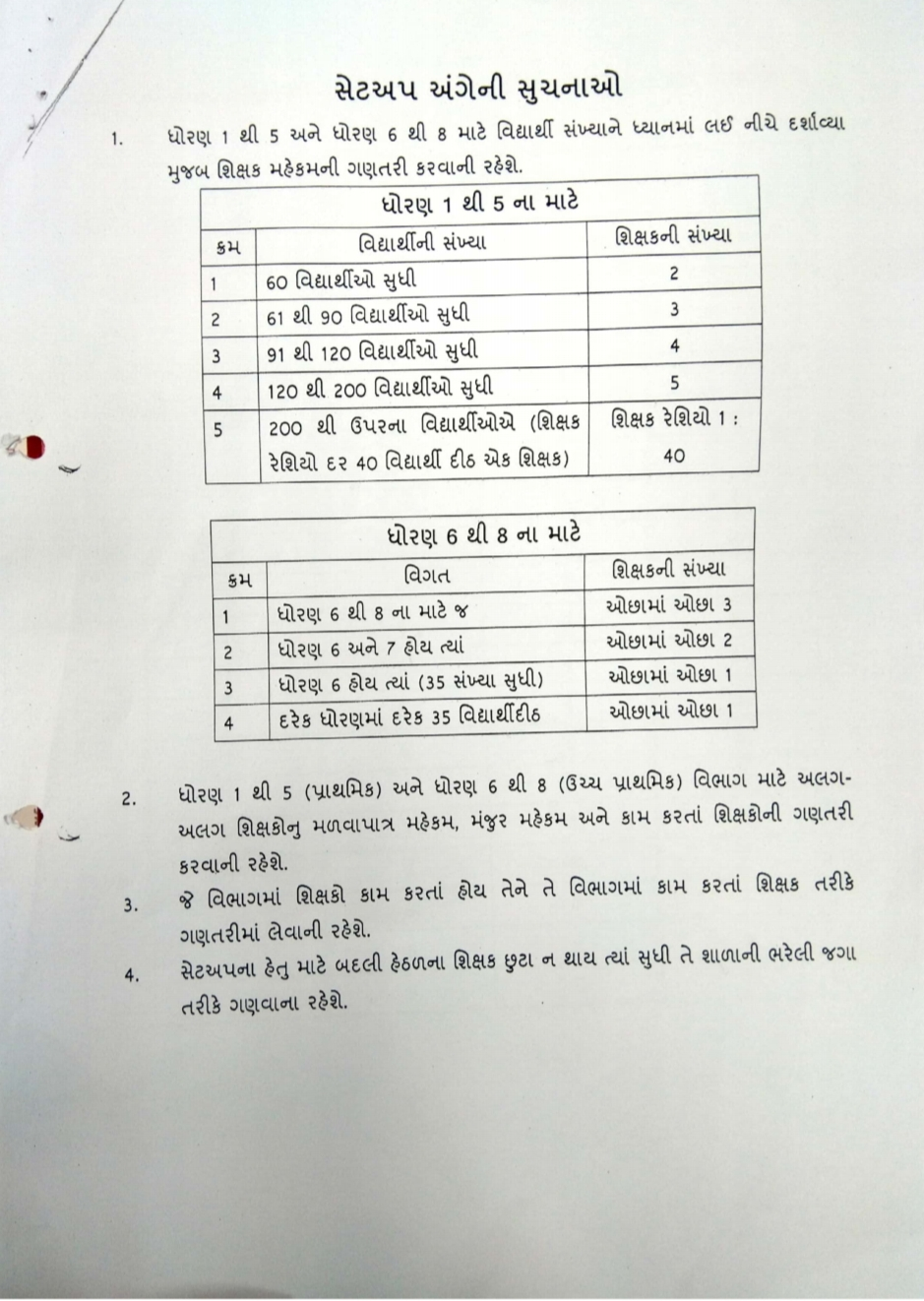## Monday, November 30, 2020

### Valuable circular dated concerning student/teacher standard set up in primary schools

Valuable circular dated concerning  student/teacher standard set up in primary schools

1, Instructions on setup The teacher establishment should be calculated as given below considering the number of students for Std. 1 to 5 and Std. 6 to 8. Rank for standard 1 to 5 Number of students Number of teachers 1 60 students 2 2 61 to 90 students 13 91 to 120 students 4. 120 to 200 students 5. S

above 200 (Teacher-Teacher Ratio 1: Ratio per 40 students one teacher) 40 3 Rank Details for Std. 6th to 8th Number of Teachers 1 | For Std. 6 to 8 only there is at least 3 2 Std. 6 and 7 where there is at least 2 3 Std. 6 where (up to number) there is at least 1 for every 35 students in each standard at least 1 2. 3. For